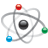# PPL and PIT Confusion

cancel
Showing results for
Did you mean:
SOLVED

## PPL and PIT Confusion

556 ViewsContributor V

I am confused with the PPL and PIT calculations.

I am using the S12XE PPL Calculator (ver 1.03)

1. Is the "Oscillator" the external 16 mHz crystal I am using.

2. Is the "Bus frequency" the frequency all timers are based on.

3. What is the "VCO frequency" and when do I use it.

4. What is the "REFCLK frequency" and when do I use it.

When using the PPL calculator to set the bus frequency to 20 mHz. This gives me 0x44 0x81 0x01 with my 16 mHz crystal ?

Now the PIT timer settings. I look at  example code and it has this example,

//fbus 8MHz

//period = (PITMTLD+1)*(PITLD+1)/fbus

//period = (19999+1)*(199+1)/8MHz = 500ms

This is confusing. I calculate the result as 0.5 not 500.

So does this formula give you the time in seconds or mSec.

I want the PIT timer to interrupt every 10 mSec. Using the same formula,

//fbus 20MHz

//period = (PITMTLD+1)*(PITLD+1)/fbus

//period = (4999+1)*(39+1)/20MHz = 0.01

if the 0.01 is seconds then 0.01 is the 10 mSec I require. The problem is the timer interrupts much faster then this.

Sorry for the silly questions. I just am new to the Freescale S12XE jargon and methods.

Ray.

Labels (1)
• ### General

1 Solution
317 ViewsNXP Employee

Hi Ray,

1. and 2. - Yes, correct.

3. and 4. - in this post you can download the PDF for the calculatorS12X PLL (Filter) Calculator.

PIT settings:

the time is in [ms]. So put 10 instead of 0.01 in you case and it should work as required.

Regards,

iggi

2 Replies
318 ViewsNXP Employee

Hi Ray,

1. and 2. - Yes, correct.

3. and 4. - in this post you can download the PDF for the calculatorS12X PLL (Filter) Calculator.

PIT settings:

the time is in [ms]. So put 10 instead of 0.01 in you case and it should work as required.

Regards,

iggi

317 ViewsContributor V

Iggi,

Thank you for the reply. I decided to use my oscilloscope to check the PIT interrupt rather then me looking at a flashing LED. The PIT is interrupting correctly. There was never a problem, only my judgment of time.

Ray.